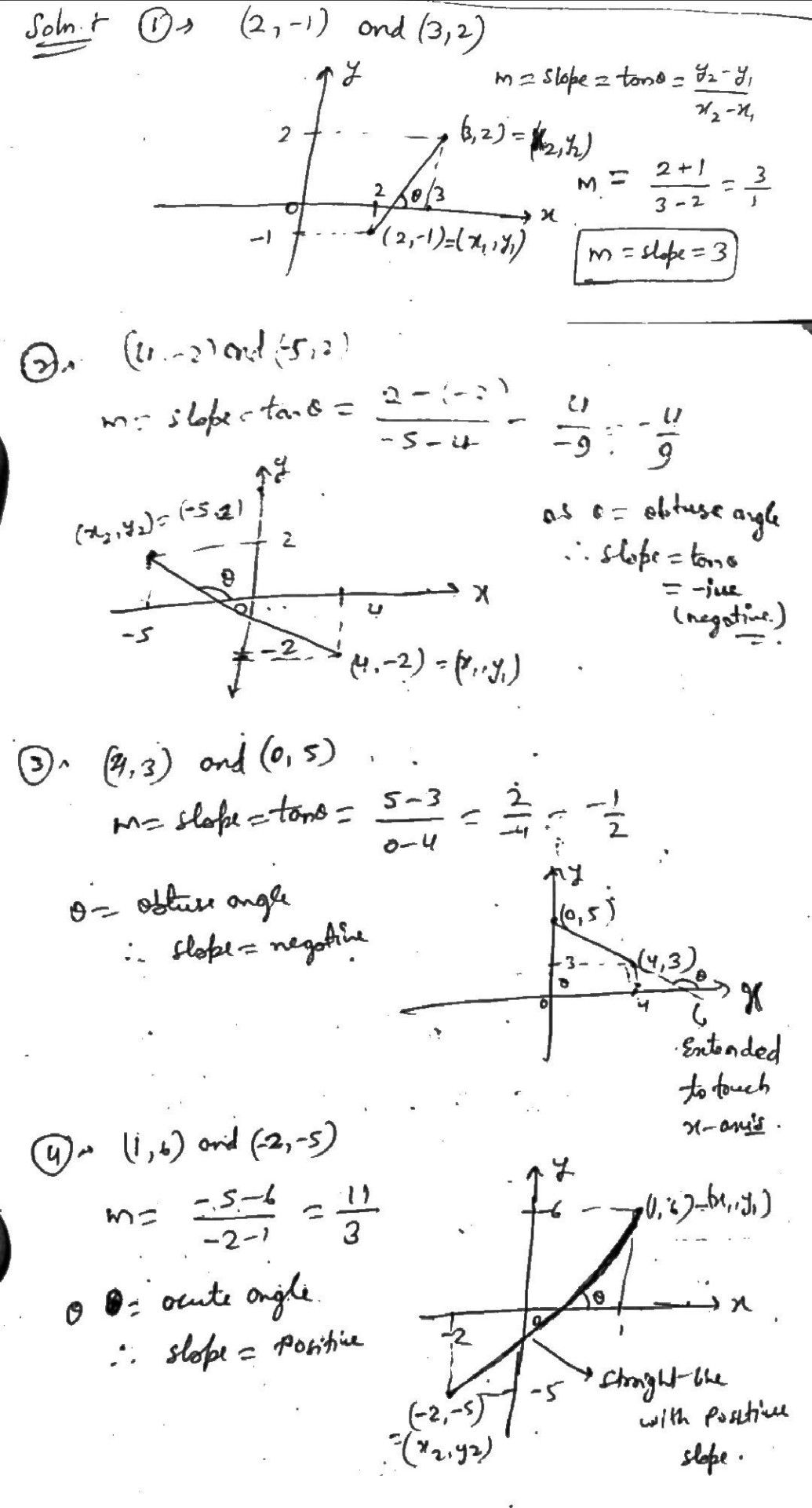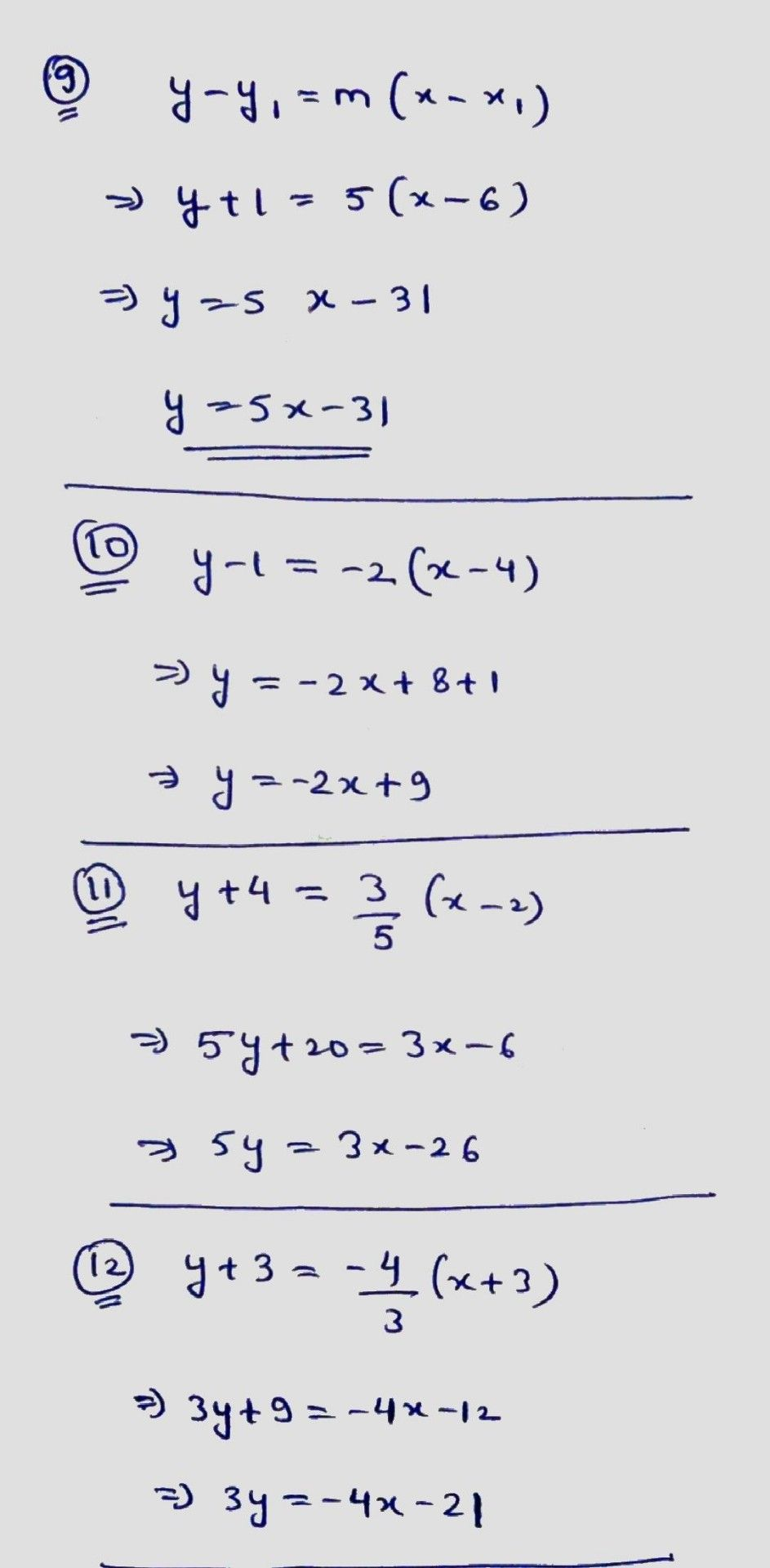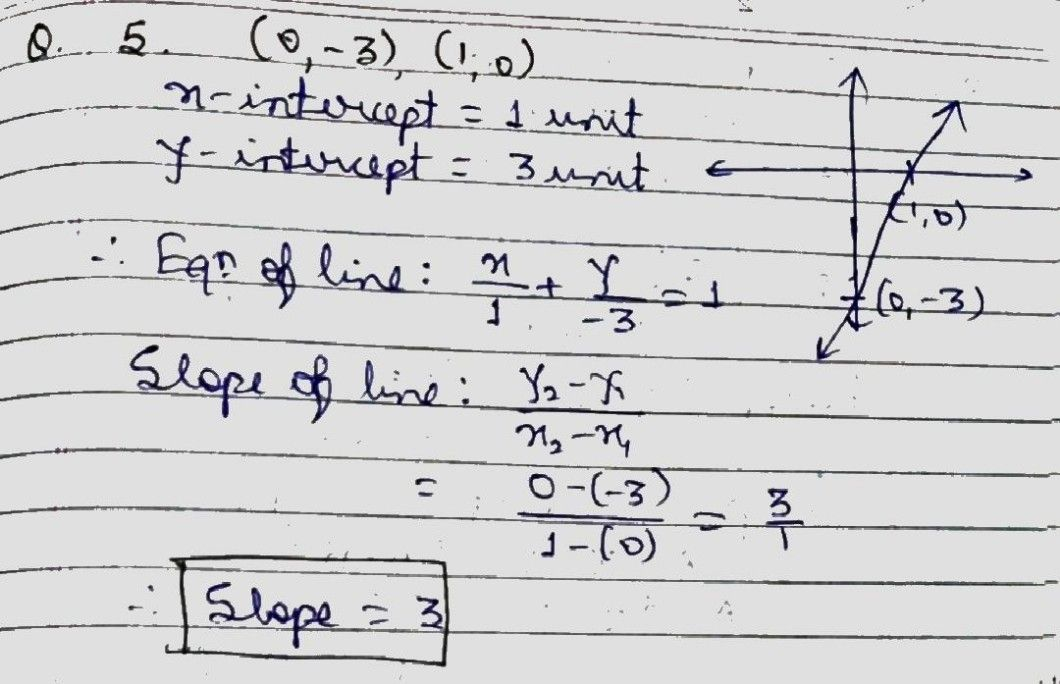Symbol
ProblemE raph the Iinear equations given the following detaila A. Using two points. 1. (2, -1) and (3, 2) 3. (4, 3) and (0, 5) 2. (4, -2) and (-5, 2) $4.$ $\left(1,6\right)$ and (-2, -5) Find the slope of cach line, Describe the graph. B. Using intercepts 5. $\left(0,$ -3) and $\left(1,0\right)$ $7.$ (5, 0) and (0, -4) 6. $\left(-3,$ 0) and $\left(0,-3\right)$ 8. $\left(2.0\right)and$ $\left(0,0,$ -4) Find the slope of the line. Describe the graph. C. Using slope and a point - and $\left(4,1\right)$ 9. $m=5$ and $\left(6,-1\right)$ 11. $m=-2a$ 10. $=3^{ana}$ $\left(2,$ -4) $12.m=$ gand $\left(-3,-3\right)$ $m=\dfrac {3} {5}$ $-\dfrac {4} {3}^{an}$
7th-9th grade
Other
Search count: 114
Question content
Can you help me?
SolutionQanda teacher - AskShivaniIf you have any doubt feel free to ask:) Dear, Please evaluate the answer and give gift coins(it does not reduce your coin balance.) for giving gift coins Click on evaluate. Then press the + icon 3 times(3000).
Dear, Please evaluate the answer and give gift coins(it does not reduce your coin balance.) for giving gift coins Click on evaluate. Then press the + icon 3 times(3000).